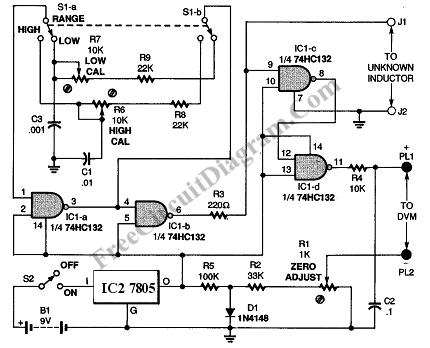# Inductance Meter CircuitThis inductance meter is actually an adapter to your digital voltmeter (DVM). Using this circuit now your voltmeter is capable of measuring inductor value. Inductance meter is very helpful in designing switch mode power supply, since its often needed  to wind the coil by hand and measure the inductance fro trial and error to get the required value. Here is the schematic diagram of the inductance meter adapter circuit: